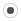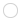## Optimizing Computationa1re
Registered MemberPosts
16
Karma
0

### Optimizing Computation

Mon May 09, 2011 5:07 pm
Good Day,

I've created the vectors a, b, c, and d (using VectorXd) and the variable 't' of type double. I'm still new to the Eigen library. What is the most optimal way that I can perform the following computation?

For the ith element, I perform
a(i) = b(i)*exp((c(i) - 1)*log(d(i)/t) - d(i))

(so it's more or less coefficient wise operations)

Also, since I'm using coefficient wise operations, do you suggest I use Eigen ArrayXd instead of VectorXd?

Thank You Very Much.ggael
ModeratorPosts
3447
Karma
19
OS### Re: Optimizing Computation

Mon May 09, 2011 9:04 pm
yes use ArrayXd and then rm the (i) from your expression, and you're doneusing namespace std;
a = b*exp((c-1)*log(d/t)-d)a1re
Registered MemberPosts
16
Karma
0

### Re: Optimizing Computation

Tue May 10, 2011 3:55 am

I've rewritten the code in terms of ArrayXd, and I have two more questions. Would there be a loss of precision when using ArrayXd compared to my previous method (where I iterate through the vector and perform the computation)? I'm obtaining slightly different estimates and since I'm writing an optimization algorithm, I cannot tell which is more accurate.

My second question is if I have vectors 'a' (a row vector) and 'b' (a column vector), what's the best way to do the following computation?

(b - 2)*(a - 1)

1) 'b - 2' should subtract 2 from 'b' coefficient wise.
2) 'a - 1' should subtract 1 from 'a' coefficient wise.
3) The resulting product should be a matrix, as I would like to perform matrix multiplication.

So far, I can achieve it in the following way:

// Create two matrices 'a' and 'b' of type MatrixXd
// 'b' is of dimension 'M x 1' and 'a' is of dimension '1 x N'

// Create the following function
double find_diff(double aa, double bb){
return (aa - bb);
}

// Then I use the unaryExpr operator
Eigen::MatrixXd c = b.unaryExpr(boost::bind(std::ptr_fun(find_diff), _1, 2.0))*a.unaryExpr(boost::bind(std::ptr_fun(find_diff), _1, 1.0));

Is there any more efficient way to perform this computation?

Thank You Once Again.jitseniesen
Registered MemberPosts
204
Karma
2

### Re: Optimizing Computation

Tue May 10, 2011 8:57 am
a1re wrote:Would there be a loss of precision when using ArrayXd compared to my previous method (where I iterate through the vector and perform the computation)? I'm obtaining slightly different estimates and since I'm writing an optimization algorithm, I cannot tell which is more accurate.

No, there should not be a loss of precision. A difference on the level of round-off error is to be expected: if you use ArrayXd, the code will be vectorized, which means that it uses different assembly instructions (and, more importantly, run faster).

a1re wrote:My second question is if I have vectors 'a' (a row vector) and 'b' (a column vector), what's the best way to do the following computation?

(b - 2)*(a - 1)

1) 'b - 2' should subtract 2 from 'b' coefficient wise.
2) 'a - 1' should subtract 1 from 'a' coefficient wise.
3) The resulting product should be a matrix, as I would like to perform matrix multiplication.

So far, I can achieve it in the following way:

// Create two matrices 'a' and 'b' of type MatrixXd
// 'b' is of dimension 'M x 1' and 'a' is of dimension '1 x N'

// Create the following function
double find_diff(double aa, double bb){
return (aa - bb);
}

// Then I use the unaryExpr operator
Eigen::MatrixXd c = b.unaryExpr(boost::bind(std::ptr_fun(find_diff), _1, 2.0))*a.unaryExpr(boost::bind(std::ptr_fun(find_diff), _1, 1.0));

If at all possible, make 'a' of type RowVectorXd and 'b' of type VectorXd. You will get more efficient code by specifying at compile-time that 'a' and 'b' are vectors instead of general M x N matrices, and it also enables the compiler to catch some errors.

Another disadvantage of your method is that the subtraction is not vectorized, because you do not provide a vectorized version of findDiff. I'd use either

Eigen::MatrixXd c = (b - Eigen::VectorXd::Constant(M, 2.0)) * (a - Eigen::RowVectorXd::Constant(N, 1.0));

or

Eigen::MatrixXd c = (b.array() - 2.0).matrix() * (a.array() - 1.0).matrix();

I would expect both to be translated to the same assembly instructions and thus have the same performance, so pick whichever you find easiest to read (in the unlikely case that performance of this instruction is very important in your program, don't just take my word for it but measure it).

In the first line, the temporary constant row/column vectors are not actually constructed. In the second line, the .matrix() and .array() function calls are free.a1re
Registered MemberPosts
16
Karma
0

### Re: Optimizing Computation

Tue May 10, 2011 5:15 pm
Thank You. Just one final question. If I have a matrix and would like to normalize each row, what's the best way to do it?

The only way I can think of would be the following:

// Suppose 'a' is the matrix

for (int i = 0; i < a.rows(); i++){
a.row(i).normalize();
}

I've now realized huge increases in speed from using this library.

Thank You for your help and Thank You to the developers of this library!ggael
ModeratorPosts
3447
Karma
19
OS### Re: Optimizing Computation

Tue May 10, 2011 8:16 pm
I guess we could add a vector-wise normalize function so that you could do:

a.rowwise().normalize()

In the meantime you can also do: a = a.rowwise().norm().eval().asDiagonal().inverse() * a;
but your loop is good too.dandelin
Registered MemberPosts
6
Karma
0

### Re: Optimizing Computation

Thu Mar 07, 2013 1:33 pm
Are there any plans to add this syntax? I find myself attempting to use it quite frequently. Especially since hnormalized() does work vector-wise.ggael
ModeratorPosts
3447
Karma
19
OS### Re: Optimizing Computation

Thu Mar 07, 2013 8:19 pm
I added a bug entry so that we don't forget about it. Feel free to have a look by yourself if you want this feature as quickly as possible! (It's in src/Core/VectorwiseOp.h)

http://eigen.tuxfamily.org/bz/show_bug.cgi?id=562

## Who is online

Registered users: Bing [Bot], Google [Bot], Sogou [Bot]